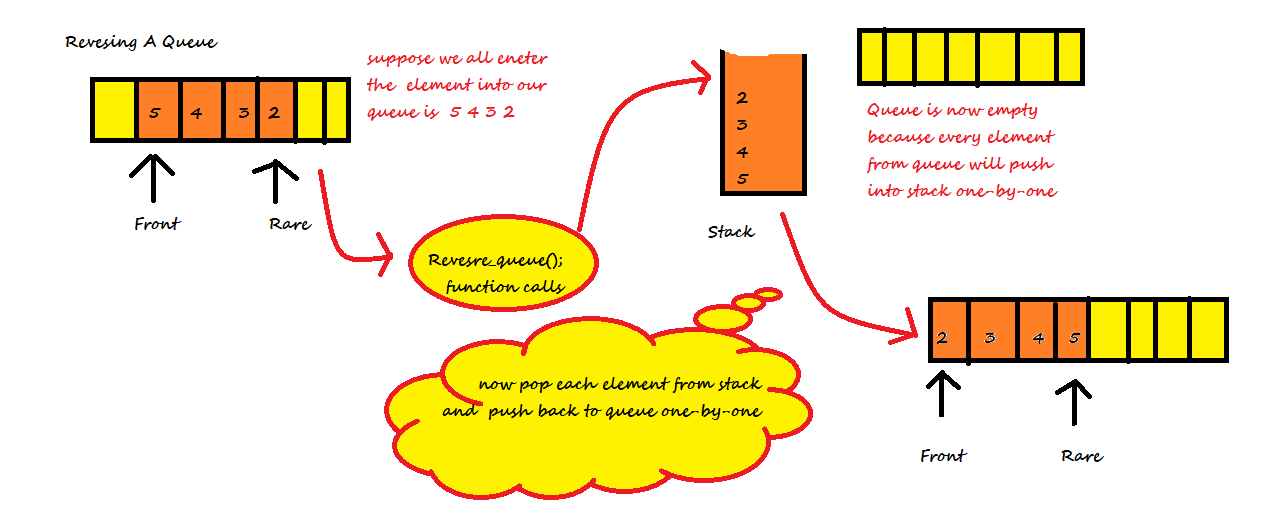×

Search anything:

# Reverse a Queue using Stack

#### Algorithms queue StackIndian Technical Authorship Contest starts on 1st July 2023. Stay tuned.

In this article, we have explored how to reverse a Queue using Stack data structure.

1. Introduction to problem statement
2. Algorithm/ Steps
3. Demonstration
4. Implementation
5. Time and Space Complexity

Pre-requisite:

### Introduction

Give an algorithm for reversing a queue Q. Only following standard operations are allowed on queue.

enqueue(x) : Add an item x to rear of queue.
dequeue() : Remove an item from front of queue.
empty() : Checks if a queue is empty or not.

### Algorithm/ Steps

Steps to reverse a Queue using Stack:

1. Enter the all elements in queue
2. Then pop all elements into stack from queue
3. At last, pop all elements from stack and push it into queue

### DemonstrationAs you can see in first step there is a queue in which elements are inserted that is 5,4,3,2 and the element 5 is at front,and element 2 is at rare.than in order to reverse the elements fist dequeue each element from queue and push it into the stack till whole queue will be empty than pop each element from the stack and enqueue it into the queue till all stack will be empty.

### Implementation

Implementation of our algorithm to reverse a Queue using Stack:

``````#include<bits/stdc++.h>
using namespace std;
stack<int> s;

void print(queue<int> &q_ueue)
{
while(!q_ueue.empty( ))
{
cout<<q_ueue.front()<<" ";
q_ueue.pop( );

}
}
void reverse(queue<int> &q_ueue)
{
while(!q_ueue.empty())
{
s.push(q_ueue.front());
q_ueue.pop();
}
while(!s.empty())
{
q_ueue.push(s.top());
s.pop();
}

}
int main( )
{

printf("enter the element in your queue to stop insertion press -1\n");
int a;
queue<int> q;
while(a!=-1)
{

cin>>a;
if(a!=-1)
{
q.push(a);
}
}
reverse(q);
print(q);

}
``````

### Time and Space Complexity

• Worst case time complexity: `Θ(O(N))`
• Average case time complexity: `Θ(O(N))`
• Best case time complexity: `Θ(O(N))`
• Space complexity: `Θ(O(N))`

## Question 1.

#### Which one of the following is an application of Stack Data Structure?

Managing function calls
The stock span problem
all of these
Arithmetic expression evaluation

## Question 2.

#### Which one of the following is an application of Queue Data Structure?

When a resource is shared among multiple consumers
When data is transferred asynchronously (data not necessarily received at same rate as sent) between two processes
all of these